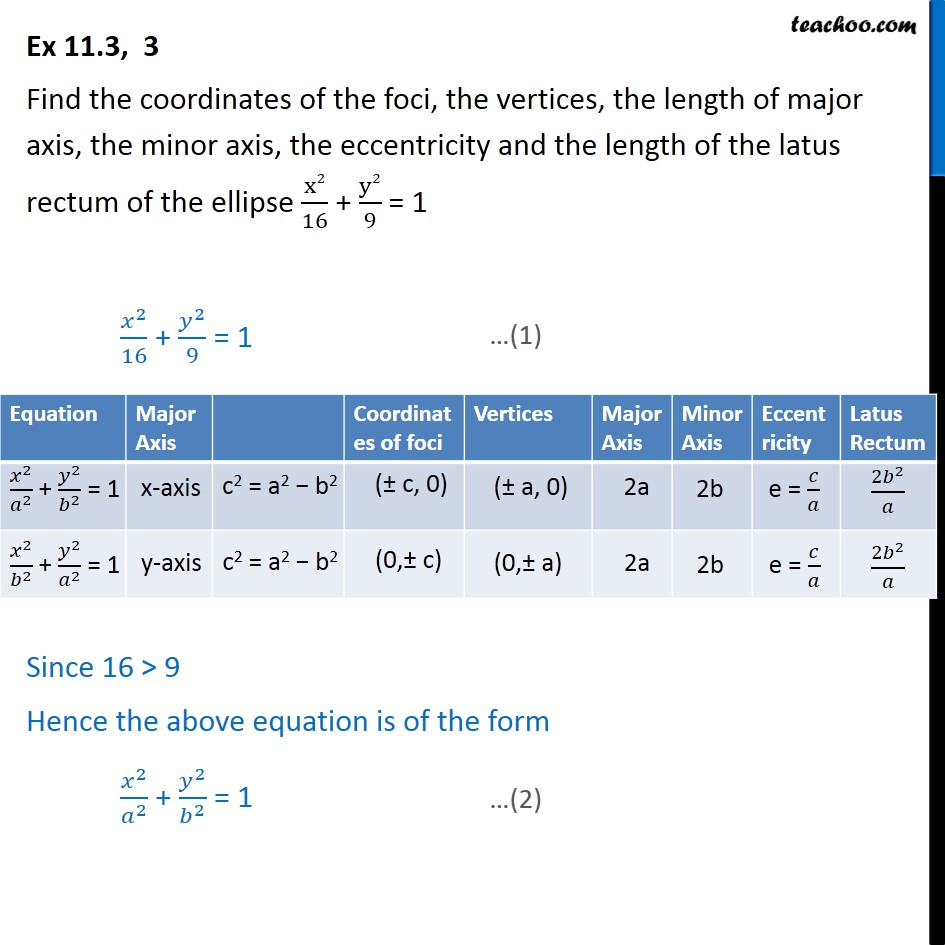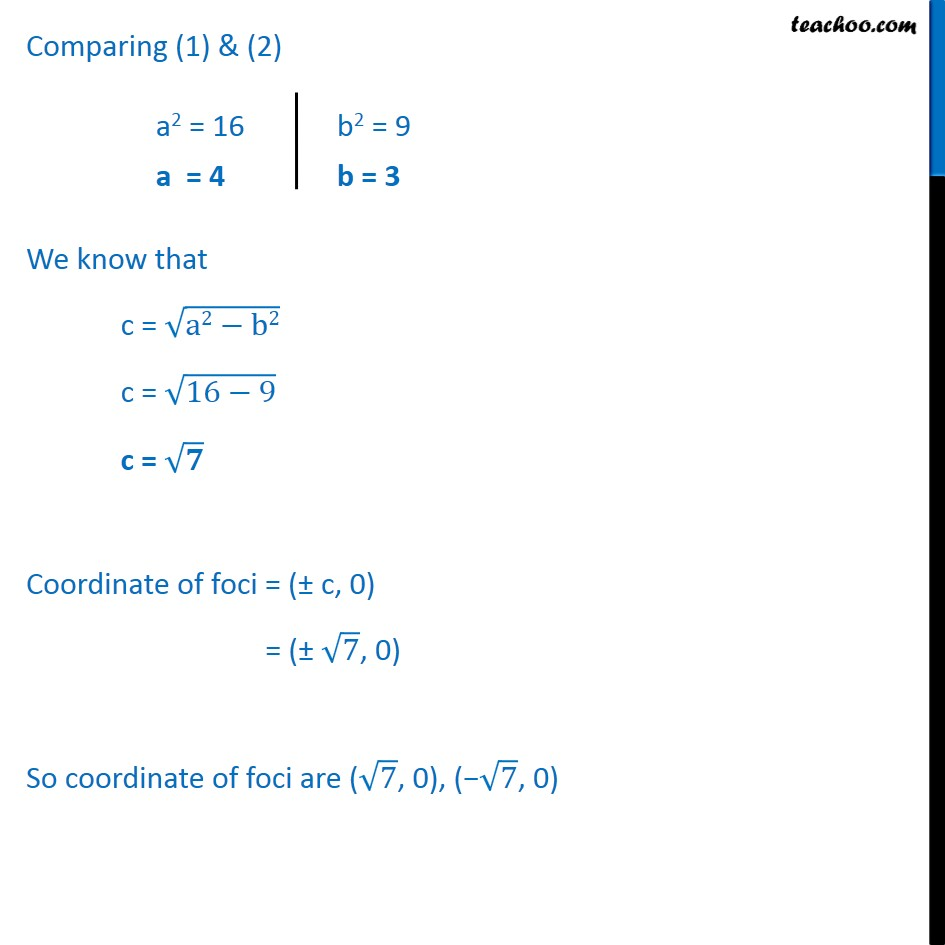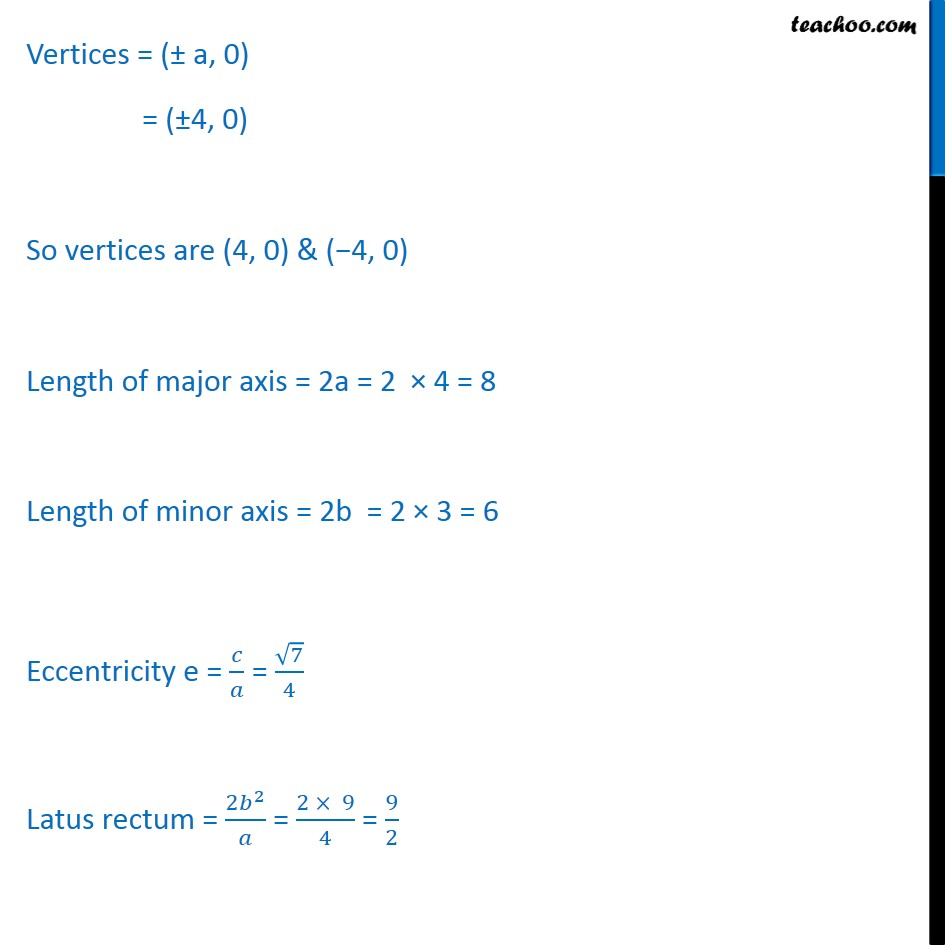Ex 10.3

Chapter 10 Class 11 Conic Sections
Serial order wiseLearn in your speed, with individual attention - Teachoo Maths 1-on-1 Class

### Transcript

Ex 10.3, 3 Find the coordinates of the foci, the vertices, the length of major axis, the minor axis, the eccentricity and the length of the latus rectum of the ellipse x2 16 + y2 9 = 1 2 16 + 2 9 = 1 Since 16 > 9 Hence the above equation is of the form 2 2 + 2 2 = 1 Comparing (1) & (2) We know that c = a2 b2 c = 16 9 c = Coordinate of foci = ( c, 0) = ( 7 , 0) So coordinate of foci are ( 7 , 0), ( 7 , 0) Vertices = ( a, 0) = ( 4, 0) So vertices are (4, 0) & ( 4, 0) Length of major axis = 2a = 2 4 = 8 Length of minor axis = 2b = 2 3 = 6 Eccentricity e = = 7 4 Latus rectum = 2 2 = 2 9 4 = 9 2